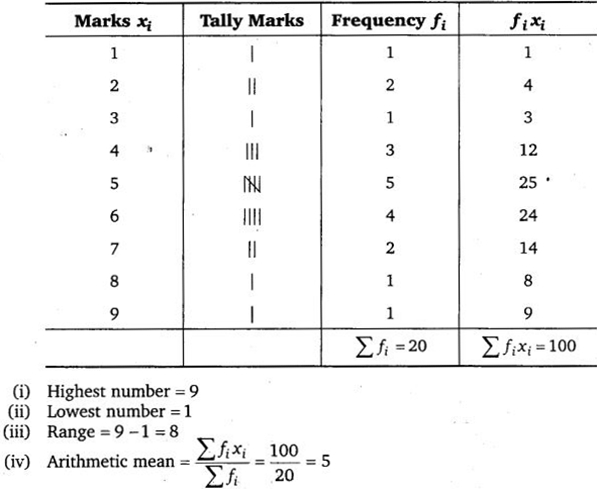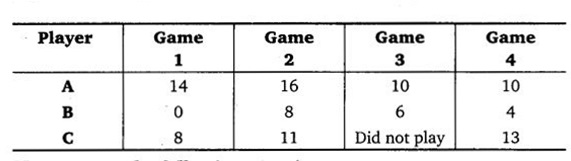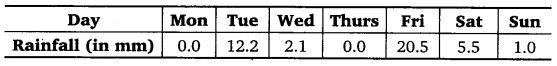# Class 7 Maths NCERT Solutions for Chapter – 3 Data Handling EX – 3.1

### Data Handling

Question 1.
Find the range of heights of any ten students of your class.

Solution:
Let the heights (in cm) of 10 students in the class be 150, 152, 151, 148, 149, 149, 150, 152, 153, 146.
Arranging the heights in ascending order, we have 146, 148, 149, 149, 150, 150, 151, 152, 152, 153.
Range of height of students = 153 – 146 = 7

Question 2.
Organise the following marks in a class assessment, in a tabular form.
4, 6, 7, 5, 3, 5, 4, 5, 2, 6, 2, 5,1, 9, 6, 5, 8, 4, 6, 7
(i) Which number is the highest?
(ii) Which number is the lowest?
(iii) What is the range of the data?
(iv) Find the arithmetic mean.

Solution:
Arranging the marks in a class assessment in a tabular form :Question 3.
Find the mean of the first five whole numbers.

Solution:
First 5 whole numbers are 0, 1, 2, 3, 4
Their mean =$\frac { 0+1+2+3+4 }{ 5 }$ =$\frac { 10 }{ 5 }$ = 2

Question 4.
A cricketer scores the following runs in eight innings: 58, 76,40, 35, 46, 45, 0,100. Find the mean score.

Solution:
Total runs = 58 + 76 + 40 + 35 + 46 + 45 + 0 +100 = 400
Number of observations = 8
∴ Mean =$\frac { 400 }{ 8 }$ = 50

Question 5.
Following table shows the points of each player scored in four games :Now answer the following questions :
(i) Find the mean to determine A’s average number of points scored per game.
(ii) To find the mean number of points per game for C, would you divide the total points by 3 or by 4 ? Why?
(iii) B played in all the four games. How would you find the mean?
(iv) Who is the best performer?

Solution:
(i) A’s average number of points scored per game$\frac { 14+16+10+10 }{ 4 }$ =$\frac { 50 }{ 4 }$ = 12.5

(ii) C’s average points per game =$\frac { 8+11+0+13 }{ 4 }$ =$\frac { 32 }{ 4 }$ = 8
Since, we are comparing the performance, so we divide by 4 to find the mean for C.

(iii) B’s average point per game =$\frac { 0+8+6+4 }{ 4 }$ =$\frac { 18 }{ 4 }$ = 4.5
(To find B’s average, we find the sum of all observations and divide this by the number of observations.)

(iv) Since, 12.5 > 8 > 4.5
∴ The best performer is A.

Question 6.
The marks (out of 100) obtained by a group of students in a science test are 85,76,90,85,39,48,56,95,81 and 75. Find the :
(i)
Highest and the lowest marks obtained by the students.
(ii) Range of the marks obtained.
(iii) Mean marks obtained by the group.

Solution:
Arranging the marks obtained by a group of students in the ascending order, we have 39, 48, 56, 75, 76, 81, 85, 85, 90 and 95.
(i) Highest and the lowest marks obtained are 95 and 39, respectively.
(ii) Range of the marks obtained = 95 – 39 =56
(iii) Mean marks

Question 7.
The enrolment in a school during six consecutive years was as follows: 1555, 1670, 1750, 2013, 2540, 2820.
Find the mean enrolment of the school for this period.

Solution:
Sum of the enrolment during six consecutive years
= 1555 + 1670 +1750 +2013 + 2540 +2820 = 12348
Mean enrolment =$\frac { 12348 }{ 6 }$ = 2058

Question 8.
The rainfall (in mm) in a city on 7 days of a certain week was recorded as follows :(i) Find the range of the rainfall in the above data.
(ii) Find the mean rainfall for the week.
(iii) On how many days was the rainfall less than the mean rainfall?

Solution:
(i) Arranging the rainfall during the week in the ascending order,
we have 0.0, 0.0, 1.0, 2.1, 5.5, 12.2, 20.5
Range = 20.5 – 0.0 = 20.5

(ii) Sum of the rainfall during the week = 0.0 + 0.0 +1.0 + 2.1 + 5.5 +12.2 + 20.5 = 41.3
Mean =$\frac { 41.3 }{ 7 }$ = 5.9

(iii) For five days, the rainfall was less than the mean rainfall.

Question 9.
The heights of 10 girls were measured in cm and the results are as follows :
135, 150, 139, 128, 151, 132, 146, 149, 143, 141.
(i) What is the height of the tallest girl?
(ii) What is the height of the shortest girl?
(iii) What is the range of the data?
(iv) What is the mean height of the girls?
(v) How many girls have heights more than the mean height?

Solution:
Arranging the heights (in cm) in the ascending order, we have 128, 132, 135, 139, 141, 143, 146, 149, 150, 151.
(i) Height of the tallest girl is 151 cm.
(ii) Height of the shortest girl is 128 cm.
(iii) Range = (151 – 128) cm = 23 cm.